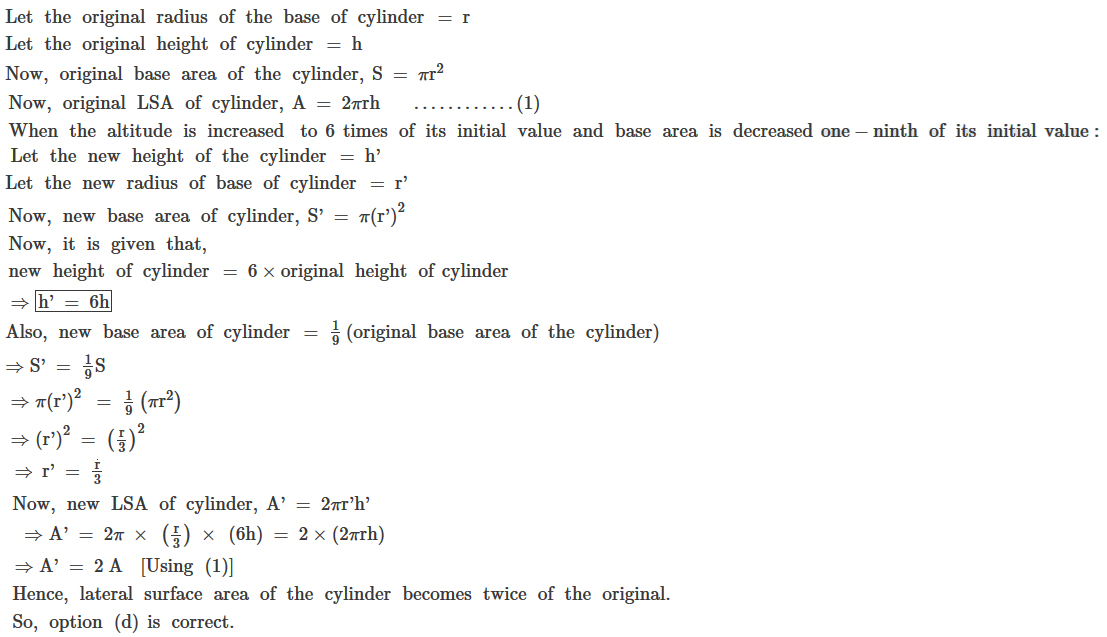#### Chapter 19 Surface Area and Volume of a Right Circular Cylinder R.D. Sharma Solutions for Class 9th MCQ's

Multiple Choice Questions

1. In a cylinder, if radius is doubled and height is halved, curved surface area will be
(a) Halved
(b) Doubled
(c) Same
(d) Four times

Solution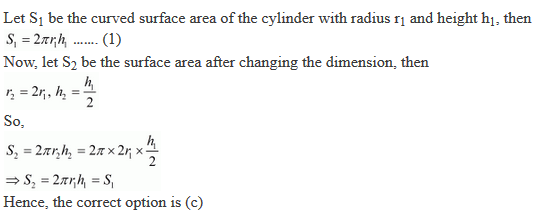2. Two cylindrical jars have their diameters in the ratio 3 : 1, but height 1 : 3. Then the ratio of their volumes is
(a) 1 : 4
(b) 1 : 3
(c) 3 : 1
(d) 2 : 5

Solution3. The number of surfaces in right cylinder is
(a) 1
(b) 2
(c) 3
(d) 4

Solution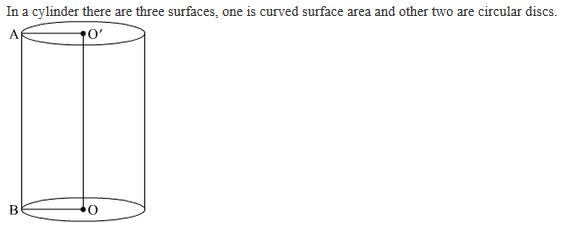4. Vertical cross-section of a right circular cylinder is always a
(a) Square
(b) Rectangle
(c) Rhombus
(d) Trapezium

Solution5. If r is the radius and h is height of the cylinder, the volume will be
(a) 1/3 πr2h
(b) πr2h
(c) 2πr (h+r)
(d) 2πrh

Solution

It is given that r is the radius and h is the height of the cylinder. We know that the cylinder is given  by the formula:
V = πr2h

6. The number of surfaces of a hollow cylindrical object is
(a) 1
(b) 2
(c) 3
(d) 4

Solution7. If the radius of a cylinder is doubled and the height remains same, the volume will be
(a) doubled
(b) halved
(c) same
(d) four times

Solution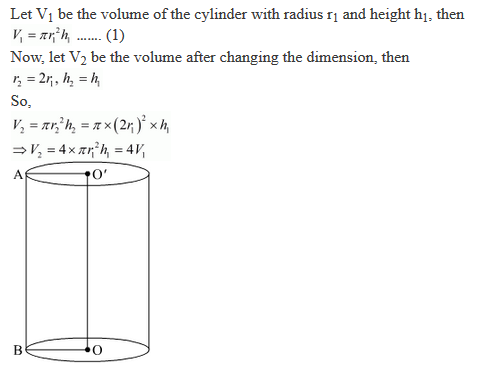8. If the height of a cylinder is doubled and radius remains the same, then volume will be
(a) doubled
(b) halved
(c) same
(d) four times

Solution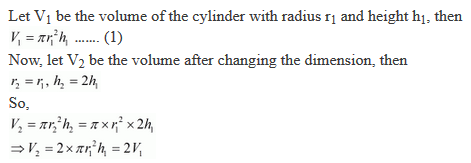9. In a cylinder, if radius is halved and height is doubled, the volume will be
(a) same
(b) doubled
(c) halved
(d) four times

Solution10. If the height of a cylinder is doubled by what number must the radius of the base be multiplied so that the resulting cylinder has the same volume as the original cylinder?

(a) 4
(b) 1/√2
(c) 2
(d) 1/2

Solution11. The volume of a cylinder of radius r is 1/4 of the volume of a rectangular box with a square of side length x. If the cylinder and the box have equal heights, what is r in terms of x?
(a) x2/2π
(b) x/2√π
(c) √2x/π
(d) π/2√x

Solution12. The height h of a cylinder equals the circumference of the cyclinder.In terms of h what is the volume of the cyclinder ?

Solution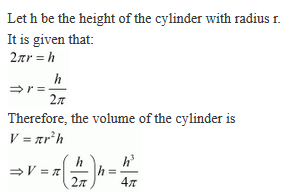13. A cylinder with radius r and height h is closed on the top and bottom. Which of the following expressions represents the total surface area of this cylinder?
(a) 2πr (r+h)
(b) πr (r+2h)
(c) πr + (2r+h)
(d) 2πr2 + h

Solution

Let S be the total surface area of the closed cylinder with radius r and height h, then
S = 2πr2 + 2πrh
⇒ S = 2πr(r+h)

14. The height of sand in a cylindrical shaped can drops 3 inches when 1 cubic foot of sand is poured out. What is the diameter in inches of the cylinder?
(a) 24/π
(b) 48/π
(c) 32/π
(d) 48/√π

Solution15.  If the diameter of the base of a closed right circular cylinder be equal to its height h, then its whole surface area is:
(a) 2πh2
(b) 3/2 πh2
(c) 4/3 πh2
(d) πh2

Solution16. A right circular cylindrical tunnel of diameter 2 m and length 40 m is to be constructed from a sheet iron. The area of the iron sheet required in m2 is:
(a) 40 π
(b) 80 π
(c) 160 π
(d) 200 π

Solution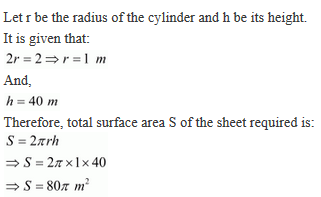17. Two steel sheets each of length a1 and breadth a2 are used to prepare the surfaces of two right circular cylinders, one have volume Vand height a2 and other having volume V2 and height a1. Then,
(a) V= V2
(b) a1V= a2V2
(c) a2V= a1V2
(d) V1/a1= V2/a2

Solution18. Two circular cylinders of equal volume have their in the ratio 1:2. Ratio of their radii is
(a) 1 : √2
(b) √2 : 1
(c) 1:2
(d) 1:4

Solution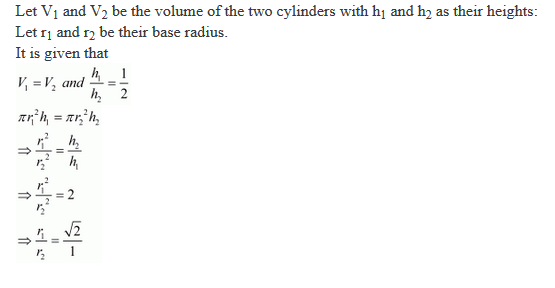19. The radius of a wire is decreased to one - third . If volume remains the same, the length will become
(a) 3 times
(b) 6 times
(c) 9 times
(d) 27 times

Solution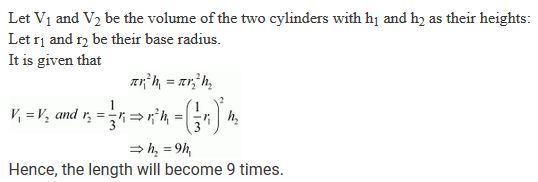20. The altitude of a circular cylinder is increased six times and the base area is decreased one-ninth of its value. The factor by which the lateral surface of the cylinder increase is
(a) 2/3
(b) 1/2
(c) 3/2
(d) 2

Solution